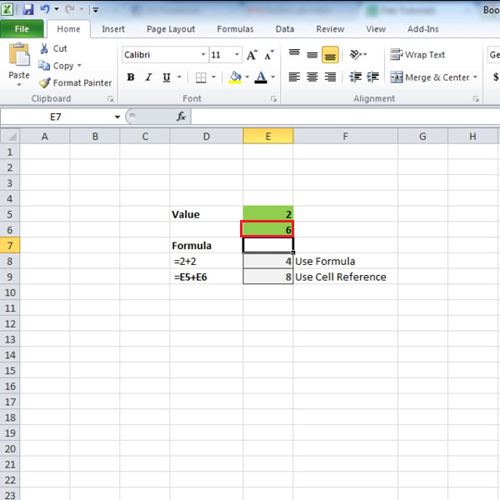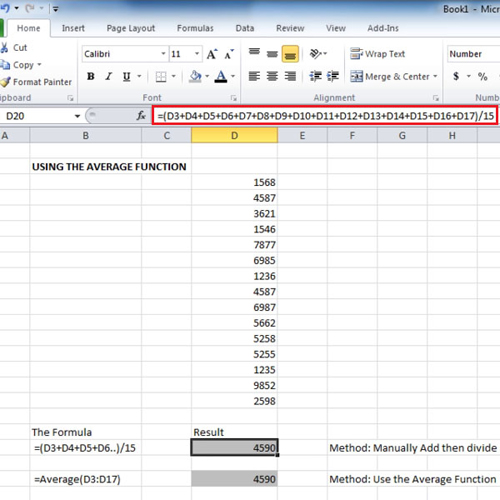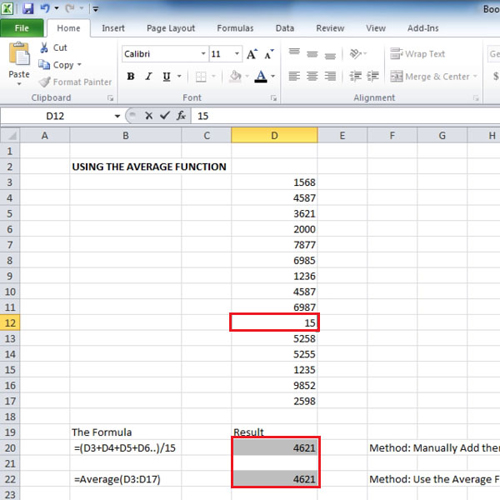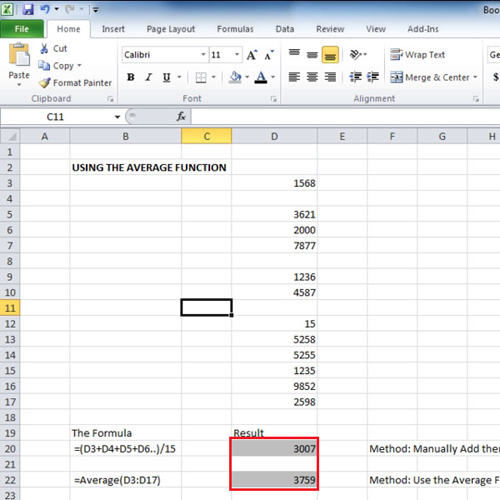## How to Understand Formulas and Functions in Excel

Microsoft Excel allows you to do calculations using formulas and functions. With the use of these formulas in excel you can easily calculate anything. There are some built in functions that you can use or you can use your own formulas as well. There are over three hundred functions in Excel. A function is a pre made formula so you can directly enter it by writing the name of the function and the rest of the formula will be showed to you automatically.

To learn in Microsoft Excel to how to use formulas and functions follow the tutorial below.

Step # 1 – Use Cell reference

There are two ways of calculating the sum. In this tutorial we have showed both of them. The first one, which is the wrong way, to calculate the sum of 2 and 2 is to write “=2+2” in a cell. This will give you the right answer however you will have to change the value in the formula each time if you want to change the value which is being calculated.
The other way is to use cell reference, this will allow you to only change the value in the cell and the sum will be calculated accordingly. Since the values are in cell “e5” and “e6” we will use the cell reference by writing “=E5+E6”. This means that we want excel to calculate the sum of whatever value is there in these cells. Over here when we changed the value of cell “E6” from “2” to “6” the answer changed from “4” to “8”. This is the correct answer.Step # 2 – Calculate the average

There are two ways of taking out the average. First is by writing each cell number in the formula. For this write:
“=(d3+d4+d5+d6+d7+d8+d9+d10+d11+d12+d13+d14+d15+d16+d17)/15”.
This basically means that excel will add all the values that are in the mentioned cells and divide them by 15 as there are 15 values. This calculation can cause some errors which we will show you later.
The other method is by using the average function. For this, write “=average(d3:d17)”.Step # 3 – Change some values

By using the two methods we can see that both show the same result although they use different techniques in calculating the averages. While learning in Microsoft Excel to how to use formulas and functions it is important to know which one should be applied where. However, if right now we change the value in cell “d6” and cell “d12”, the answer still comes out to be same.Step # 4 – Delete some values

Up till now the result was the same but as you start deleting some of the values you will notice that the first method will show a result which will be lower in value. This is because the first method is still dividing the sum of all the values by “15”, which it shouldn’t. It should only divide the values by the total number of cells which have values in them. The second method, instead of dividing it by “15” is dividing it by only “12” as we have deleted values of 3 cells. Therefore it is important to know which formulas in excel you should use so as to get accurate results.